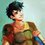# What's a Vector in Mathematics?

I'm trying to learn about Vectors in Math, but I can't find a website where I can understand anything, please help me understand by posting a good link, or a good explanation of what a vector in math is..............Note by Percy Jackson
6 months ago

This discussion board is a place to discuss our Daily Challenges and the math and science related to those challenges. Explanations are more than just a solution — they should explain the steps and thinking strategies that you used to obtain the solution. Comments should further the discussion of math and science.

When posting on Brilliant:

• Use the emojis to react to an explanation, whether you're congratulating a job well done , or just really confused .
• Ask specific questions about the challenge or the steps in somebody's explanation. Well-posed questions can add a lot to the discussion, but posting "I don't understand!" doesn't help anyone.
• Try to contribute something new to the discussion, whether it is an extension, generalization or other idea related to the challenge.

MarkdownAppears as
*italics* or _italics_ italics
**bold** or __bold__ bold
- bulleted- list
• bulleted
• list
1. numbered2. list
1. numbered
2. list
Note: you must add a full line of space before and after lists for them to show up correctly
paragraph 1paragraph 2

paragraph 1

paragraph 2

[example link](https://brilliant.org)example link
> This is a quote
This is a quote
    # I indented these lines
# 4 spaces, and now they show
# up as a code block.

print "hello world"
# I indented these lines
# 4 spaces, and now they show
# up as a code block.

print "hello world"
MathAppears as
Remember to wrap math in $$ ... $$ or $ ... $ to ensure proper formatting.
2 \times 3 $2 \times 3$
2^{34} $2^{34}$
a_{i-1} $a_{i-1}$
\frac{2}{3} $\frac{2}{3}$
\sqrt{2} $\sqrt{2}$
\sum_{i=1}^3 $\sum_{i=1}^3$
\sin \theta $\sin \theta$
\boxed{123} $\boxed{123}$

Sort by:

Hi Percy. This is a really amazing "interactive textbook" all about linear algebra. Hope you find it helpful!

- 6 months ago

Thanks David!

- 5 months, 3 weeks ago

And a vector $\vec{z}$ (\vec{z}) is represented like this: $(a,b)$, which denotes if you put its starting point on the origin, it’s endpoint is on $(a,b)$.

- 6 months ago

Doesn't the way you represent vectors differ from country to country? E.g., I've always learnt to write vectors as $\begin{pmatrix} a \\ b \end{pmatrix}$.

- 5 months, 4 weeks ago

$\begin{pmatrix} a \ b \end{pmatrix}$ - YOu mean this @Lorenz W.

- 5 months, 4 weeks ago

No, in a way where a is located on the top of b.

- 5 months, 4 weeks ago

$\begin{pmatrix} \frac{a}{b} \end{pmatrix}$

- 5 months, 4 weeks ago

Yes kind of like that, if you'd remove the linke between a and b )

- 5 months, 4 weeks ago

$\begin{pmatrix} a \\ b \end{pmatrix}$ @Lorenz W. put double \ after a instead of \ in your latex :)

- 5 months, 4 weeks ago

How did you do that in Latex?

- 5 months, 4 weeks ago

Just a \choose b $a\choose b$

- 5 months, 4 weeks ago

\s(s\begin{pmatrix} a \s\ b \end{pmatrix} \s) but kick all the s out

- 5 months, 4 weeks ago

@Lorenz W. put double \ after a instead of \ in your latex :)

- 5 months, 4 weeks ago

Ok. Thank you

- 5 months, 4 weeks ago

A vector would be used to show the distance and direction something moved in. If you ask for directions, and a person says "Walk one kilometer towards the North", that's a vector. If he says "Walk one kilometer", without showing a direction, it would be a scalar.

@Percy Jackson, this is for you...

- 6 months ago

That is physics, where a vector has both direction and magnitude, I'm talking about vectors in Math, as in Euclidian geometry and Euclidian vectors @Hamza Anushath, thanks for trying!

- 6 months ago

But we draw them on diagrams instead...

- 6 months ago

@Percy Jackson, they are the same thing! Check it out yourself...

- 6 months ago

Oh! Thanks, I thought that they were different things, as it was in in the 12th std Syllabus of maths. @Hamza Anushath

- 6 months ago